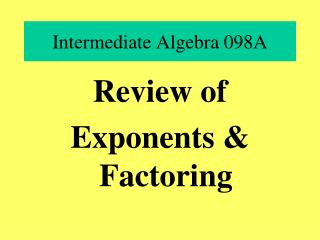DownloadDownload PresentationIntermediate Algebra 098A

# Intermediate Algebra 098A

Télécharger la présentation## Intermediate Algebra 098A

- - - - - - - - - - - - - - - - - - - - - - - - - - - E N D - - - - - - - - - - - - - - - - - - - - - - - - - - -
##### Presentation Transcript

1. Intermediate Algebra 098A Review of Exponents & Factoring

2. 1.1 – Integer Exponents • For any real number b and any natural number n, the nth power of b is found by multiplying b as a factor n times.

3. Exponential Expression – an expression that involves exponents • Base – the number being multiplied • Exponent – the number of factors of the base.

4. Product Rule

5. Quotient Rule

6. Integer Exponent

7. Zero as an exponent

8. Calculator Key • Exponent Key

9. Sample problem

10. more exponents • Power to a Power

11. Product to a Power

12. Polynomials - Review • Addition • and • Subtraction

13. Objective: • Determine the coefficient and degree of a monomial

14. Def: Monomial • An expression that is a constant or a product of a constant and variables that are raised to whole –number powers. • Ex: 4x 1.6 2xyz

15. Definitions: • Coefficient: The numerical factor in a monomial • Degree of a Monomial: The sum of the exponents of all variables in the monomial.

16. Examples – identify the degree

17. Def: Polynomial: • A monomial or an expression that can be written as a sum or monomials.

18. Def: Polynomial in one variable: • A polynomial in which every variable term has the same variable.

19. Definitions: • Binomial: A polynomial containing two terms. • Trinomial: A polynomial containing three terms.

20. Degree of a Polynomial • The greatest degree of any of the terms in the polynomial.

21. Examples:

22. Objective • Add • and • Subtract • Polynomials

23. To add or subtract Polynomials • Combine Like Terms • May be done with columns or horizontally • When subtracting- change the sign and add

24. Evaluate Polynomial Functions • Use functional notation to give a polynomial a name such as p or q and use functional notation such as p(x) • Can use Calculator

25. Calculator Methods • 1. Plug In • 2. Use [Table] • 3. Use program EVALUATE • 4. Use [STO->] • 5. Use [VARS] [Y=] • 6. Use graph- [CAL][Value]

26. Objective: • Apply evaluation of polynomials to real-life applications.

27. Intermediate Algebra 5.4 • Multiplication • and • Special Products

28. Objective • Multiply • a • polynomial • by a • monomial

29. Procedure: Multiply a polynomial by a monomial • Use the distributive property to multiply each term in the polynomial by the monomial. • Helpful to multiply the coefficients first, then the variables in alphabetical order.

30. Law of Exponents

31. Objectives: • Multiply Polynomials • Multiply Binomials. • Multiply Special Products.

32. Procedure: Multiplying Polynomials • 1. Multiply every term in the first polynomial by every term in the second polynomial. • 2. Combine like terms. • 3. Can be done horizontally or vertically.

33. Multiplying Binomials • FOIL • First • Outer • Inner • Last

34. Product of the sum and difference of the same two termsAlso called multiplying conjugates

35. Squaring a Binomial

36. Objective: • Simplify Expressions • Use techniques as part of a larger simplification problem.

37. Albert Einstein-Physicist • “In the middle of difficulty lies opportunity.”

38. Intermediate Algebra –098A • Common Factors • and • Grouping

39. Def: Factored Form • A number or expression written as a product of factors.

40. Greatest Common Factor (GCF) • Of two numbers a and b is the largest integer that is a factor of both a and b.

41. Calculator and gcd • [MATH][NUM]gcd( • Can do two numbers – input with commas and ). • Example: gcd(36,48)=12

42. Greatest Common Factor (GCF) of a set of terms • Always do this FIRST!

43. Procedure: Determine greatest common factor GCF of 2 or more monomials • 1. Determine GCF of numerical coefficients. • 2. Determine the smallest exponent of each exponential factor whose base is common to the monomials. Write base with that exponent. • 3. Product of 1 and 2 is GCF

44. Factoring Common Factor • 1. Find the GCF of the terms • 2. Factor each term with the GCF as one factor. • 3. Apply distributive property to factor the polynomial

45. Example of Common Factor

46. Factoring when first terms is negative • Prefer the first term inside parentheses to be positive. Factor out the negative of the GCF.

47. Factoring when GCF is a polynomial

48. Factoring by Grouping – 4 terms • 1. Check for a common factor • 2. Group the terms so each group has a common factor. • 3. Factor out the GCF in each group. • 4. Factor out the common binomial factor – if none , rearrange polynomial • 5. Check

49. Example – factor by grouping

50. Ralph Waldo Emerson – U.S. essayist, poet, philosopher • “We live in succession , in division, in parts, in particles.”